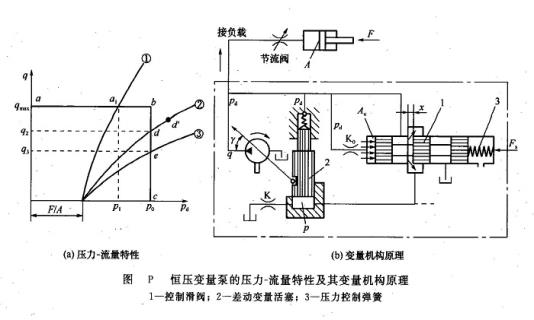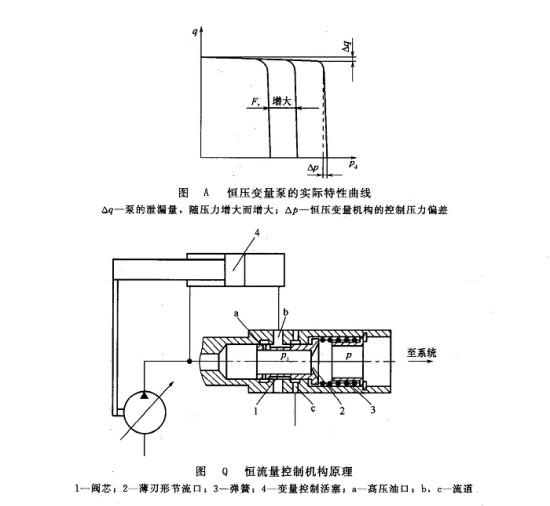Created on：2021-05-31 11:45

Constant pressure control variable and constant flow control

Constant pressure control variable and constant flow control

b. The constant pressure variable mechanism in the constant pressure variable pump regulates the output flow of the pump through the difference between the pump outlet pressure and the set value of the variable mechanism pressure, so as to keep the pump outlet pressure at the set value. This pump is a constant displacement pump before the system pressure reaches the set value, which provides the maximum flow of the pump to the system; when the system pressure reaches the set value, its output pressure is constant no matter how the output flow changes, so it is called constant pressure variable pump. The pressure flow characteristic of the pump is shown in Figure P (a), and the working principle of the constant pressure variable mechanism is shown in Figure P (b). The pump outlet pressure is introduced into the left end of the pilot control spool valve 1 to form the hydraulic thrust PDAC, which is compared with the force FS of the right end pressure control spring. FS represents the given pressure P0 of constant pressure pump, that is, P0 = FS / AC.When the working pressure PD of the pump is less than P0, the opening X of the slide valve 1 is O, and the pressure P of the large diameter end of the differential variable piston 2 is 0. Driven by the oil pressure PD of the small diameter end, the piston 2 pushes the swash plate to the position of maximum γ, so as to maintain the maximum flow Qmax of the pump [the horizontal line AB in Figure P (a)]. When the working pressure of the pump increases to the given value of the pump, i.e. PD = P0, the hydraulic thrust PDAC at the left end of the slide valve 1 will overcome the spring force FS and open the valve port to form a variable orifice with an opening of X, which forms a series resistance circuit with the fixed throttle K. The resistance circuit can be used to control the big end pressure P of differential variable piston 2: when the opening x increases, the pressure P increases. When x increases to a certain extent, the pressure P can push the differential variable piston 2 to move upward and drive the swash plate, so that γ decreases and the flow of the pump decreases. Because the pilot control slide valve 1 does not directly push the swash plate, but only controls the differential variable piston 2 pushing the swash plate, the size is very small, so the stiffness of spring 3 is also very small. Therefore, when PD = P 0, the opening of control valve 1 can be arbitrary in theory, and the position of differential variable piston and swash plate angle are also arbitrary. This means that when PD = P0, the pump may work at any flow rate between q = 0 and q = Qmax [constant pressure line BC in Figure P (a)]. If the external load is too large and the pump pressure PD > P0, the pump can not work. Because when PD reaches P 0 and has a tendency to continue to rise, the opening X of control spool valve 1 has already reached the maximum, and the pressure at the big end of differential variable piston has also reached the maximum, and the swashplate is pushed to the position of γ = O to make the output flow zero. In practical application, it is necessary to use the load with throttling resistance and the constant pressure pump to work in the constant pressure area. The curves (1), (2) and (3) in Figure P (a) are the resistance flow characteristic curves of three throttling loads, which intersect the constant pressure line BC at D and P. The characteristic of throttling load is that it does not require a fixed pressure, a working pressure corresponds to a certain flow, and the flow increases with the increase of pressure. In this way, the intersection points D and e of the throttle resistance flow characteristic curve (2) and (3) and the constant pressure characteristic line (BC) of the constant pressure pump are the stable operating points. The process of forming these operating points is as follows: if the working point is disturbed and deviates, for example, the working point d moves to the point d 'along the resistance flow characteristic curve, the flow increases, and the working pressure of the pump is also higher than P0, which destroys the force balance state of the control slide valve 1, and then the flow decreases with the increase of the valve opening x, the increase of the pressure at the big end of the differential variable piston, and the decrease of the swash plate angle γ . This feedback process will continue until the operating point returns to the original d point. It can be seen that the constant pressure variable displacement pump can provide a constant pressure oil source with a pressure of P0. Figure a is the actual characteristic curve of the constant pressure variable displacement pump. The constant pressure characteristics with different pressures can be obtained by adjusting the control spring to change FS. The constant pressure variable displacement pump can be used to maintain the pressure of the hydraulic system, the output flow only compensates the system leakage; it can be used as the constant pressure oil source of the electro-hydraulic servo system; it can be used in the throttle speed control system.

If the pressure regulating mechanism is replaced by a proportional electromagnet and the control valve is an electro-hydraulic proportional valve, the electro-hydraulic proportional constant pressure control pump can be formed. The working pressure of the pump is proportional to the input control current of the proportional electromagnet.c. Constant flow control chart Q shows the principle of traditional pressure control type constant flow control mechanism. A thin blade shaped orifice 2 is set on the constant flow control valve as the flow detection element, which converts the flow change into the pressure change signal to control the position of the spool 1. When the actual output flow of the pump decreases for some reason, the differential pressure Δ P (= p1-p) of the throttle port decreases, and the elastic force of the spring 3 is greater than the hydraulic pressure, which makes the valve core 1 move to the left. Therefore, the high pressure oil from port a enters the right end of variable control piston 4 through passage B, which makes the variable mechanism move, so the displacement of the pump increases. Because of the constant flow control, when the pump works under any pressure (i.e. different volumetric efficiency), its output flow can be kept constant. For the piston pump, because of its high volumetric efficiency, when the speed is constant, in a certain precision range, constant displacement has the function of constant flow. The constant flow pump can keep the output flow constant in a certain speed range when the prime mover speed of the driving pump changes greatly (such as internal combustion engine).

Home    Article    Constant pressure control variable and constant flow control